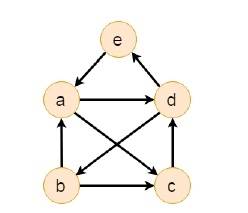# C++ Program to Check Whether a Directed Graph Contains a Eulerian Path

The Euler path is a path; by which we can visit every edge exactly once. We can use the same vertices for multiple times. One graph which contains Euler Circuit is also considered in this case, as it also has the Euler path.

To check whether a directed graph has Euler path or not, we have to check these conditions -

• There must be one single vertex an where (in-degree + 1 = out_degree)
• There must be one single vertex bn where (in-degree = out_degree + 1)
• Rest all vertices have (in-degree = out_degree) if any of these cases fails, the graph has no Euler path.Vertex b has (in-degree 1, out-degree 2), vertex c has (in-degree 2, out-degree 1). And for the rest of the vertices a, d has (in-degree 2, out-degree 2), e has (in-degree 1, out-degree 1).

## Input

 0 0 1 1 0 1 0 1 0 0 0 0 0 1 0 0 1 0 0 1 1 0 0 0 0

## Output

Euler Path Found.

## traverse(u, visited)

Input The start node u and the visited node to mark which node is visited.

Output Traverse all connected vertices.

Begin
mark u as visited
for all vertex v, if it is adjacent with u, do
if v is not visited, then
traverse(v, visited)
done
End

## isConnected(graph)

Input : The graph.

Output: True if the graph is connected.

Begin
define visited array
for all vertices u in the graph, do
make all nodes unvisited
traverse(u, visited)
if any unvisited node is still remaining, then
return false
done
return true
End

## hasEulerPath(Graph)

Input The given Graph.

Output True when one Euler circuit is found.

Begin
an := 0
bn := 0
if isConnected() is false, then
return false
define list for inward and outward edge count for each node
for all vertex i in the graph, do
sum := 0
for all vertex j which are connected with i, do
inward edges for vertex i increased
increase sum
done
number of outward of vertex i is sum
done
if inward list and outward list are same, then
return true
for all vertex i in the vertex set V, do
if inward[i] ≠ outward[i], then
if inward[i] + 1 = outward[i], then
an := an + 1
else if inward[i] = outward[i] + 1, then
bn := bn + 1
done
if an and bn both are 1, then
return true
otherwise return false
End

## Example Code

#include<iostream>
#include<vector>
#define NODE 5
using namespace std;
int graph[NODE][NODE] = {{0, 0, 1, 1, 0},
{1, 0, 1, 0, 0},
{0, 0, 0, 1, 0},
{0, 1, 0, 0, 1},
{1, 0, 0, 0, 0}};
void traverse(int u, bool visited[]) {
visited[u] = true; //mark v as visited
for(int v = 0; v<NODE; v++) {
if(graph[u][v]) {
if(!visited[v])
traverse(v, visited);
}
}
}
bool isConnected() {
bool *vis = new bool[NODE];
//for all vertex u as start point, check whether all nodes are visible or not
for(int u; u < NODE; u++) {
for(int i = 0; i<NODE; i++)
vis[i] = false; //initialize as no node is visited
traverse(u, vis);
for(int i = 0; i<NODE; i++) {
if(!vis[i]) //if there is a node, not visited by traversal, graph is not connected
return false;
}
}
return true;
}
bool hasEulerPath() {
int an = 0, bn = 0;
if(isConnected() == false){ //when graph is not connected
return false;
}
vector<int> inward(NODE, 0), outward(NODE, 0);
for(int i = 0; i<NODE; i++) {
int sum = 0;
for(int j = 0; j<NODE; j++) {
if(graph[i][j]) {
inward[j]++; //increase inward edge for destination vertex
sum++; //how many outward edge
}
}
outward[i] = sum;
}
//check the condition for Euler paths
if(inward == outward) //when number inward edges and outward edges for each node is same
return true; //Euler Circuit, it has Euler path
for(int i = 0; i<NODE; i++) {
if(inward[i] != outward[i]) {
if((inward[i] + 1 == outward[i])) {
an++;
} else if((inward[i] == outward[i] + 1)) {
bn++;
}
}
}
if(an == 1 && bn == 1) { //if there is only an, and bn, then this has euler path
return true;
}
return false;
}
int main() {
if(hasEulerPath())
cout << "Euler Path Found.";
else
cout << "There is no Euler Circuit.";
}

## Output

Euler Path Found.